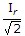# Electronics and Communication Engineering - Power Electronics

### Exercise :: Power Electronics - Section 7

36.

A cycloconverter is a group of controlled rectifiers.

 A. True B. False

Explanation:

No answer description available for this question. Let us discuss.

37.

A single phase full wave regulator feeds a resistive load. The firing angle of thyristors is 90°. The input power factor is

 A. 1 B. 0.707 lagging C. 0.5 lagging D. 0.2 lagging

Explanation:

No answer description available for this question. Let us discuss.

38.

The leads in a TRIAC are denoted by

 A. anode cathode, gate B. T1 T2, gate C. T1, T2, anode D. T1, T2, T3

Explanation:

No answer description available for this question. Let us discuss.

39.

A step down cycloconverter has an input frequency f. If it uses natural commutation, the output frequency

 A. must be a sub-multiple of input frequency B. can be any frequency C. must be an even sub-multiple of input frequency D. must be an odd sub-multiple of input frequency

Explanation:

No answer description available for this question. Let us discuss.

40.

In a single phase full wave regulator, the rms current in each thyristors is Ir The run load current is equal to

 A. 2Ir B. 2 Ir C. Ir D.